Math resources Geometry Perimeter

Perimeter of a square

# Perimeter of a square

Here you will learn how to find the perimeter of a square, including what the perimeter is, how to calculate it and how to solve perimeter word problems.

Students will first learn how to find perimeter as part of measurement and data in 3 rd grade.

## What is perimeter of a square?

The perimeter of a square is the total distance around the outside of the square.

For example,

Perimeter is measured in units. Each side of the square is one unit.

The perimeter of the square is found by counting the units on each side of the square. You can do this by starting at a vertex and counting each unit until you arrive back at the vertex.

There are 16 units around the outside of the square, so the perimeter is 16 units.

Notice the perimeter can also be calculated by adding the length of each side.

4 + 4 + 4 + 4 = 16 , so the perimeter is 16 units.

Since each side of a square is the same, you can also multiply one side length by 4 to calculate the perimeter.

4 \text { units } \times 4=16 \text { units }

The general perimeter of a square formula is \, 4 \, \times \text { side length } or \, 4s .

### What is perimeter of a square?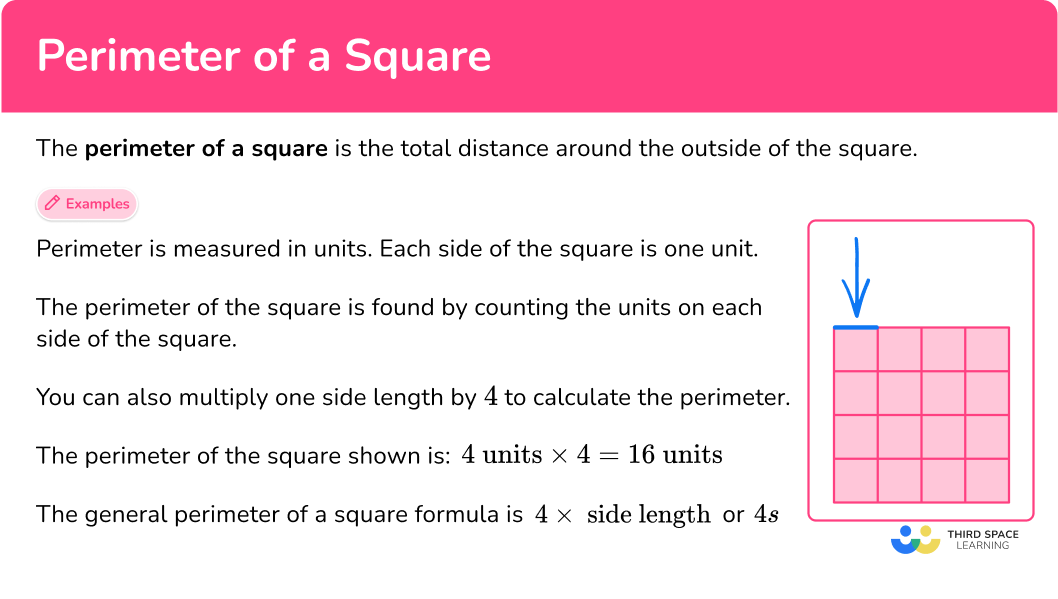## Common Core State Standards

How does this relate to 3 rd grade math?

• Grade 3 – Measurement and data (3.MD.D.8)
Solve real world and mathematical problems involving perimeters of polygons, including finding the perimeter given the side lengths, finding an unknown side length, and exhibiting rectangles with the same perimeter and different areas or with the same area and different perimeters.

## How to find the perimeter of a square

In order to calculate the perimeter of a square:

1. Add all the side lengths.
2. Write the final answer with the correct units.

## Perimeter of a square examples

### Example 1: gridded square

What is the perimeter of the square?

1. Add all the side lengths.

This side of the square is 3 units. This means every side of the square is 3 units.

To find the perimeter (the total distance around the square), add all the side lengths:

3 + 3 + 3 + 3 = 12

Since each side of a square is the same, you can also multiply one side length by 4 to calculate the perimeter: 3 \times 4=12.

2Write the final answer with the correct units.

There are no specific units given, so they are just labeled ‘units.’

The perimeter of the square is 12 units.

### Example 2: gridded square

What is the perimeter of the square?

This side of the square is 5 units. This means every side of the square is 5 units.

To find the perimeter (the total distance around the square), add all the side lengths:

5 + 5 + 5 + 5 = 20

Since each side of a square is the same, you can also multiply one side length by 4 to calculate the perimeter: 5 \times 4=20 .

There are no specific units given, so they are just labeled ‘units.’

The perimeter of the square is 20 units.

### Example 3: non-gridded square

What is the perimeter of the square?

One side of the square is 7 feet. This means every side of the square is 7 feet.

To find the perimeter (the total distance around the square), add all the side lengths:

7 + 7 + 7 + 7 = 28

Since each side of a square is the same, you can also multiply one side length by 4 to calculate the perimeter: 7 \times 4=28 .

The side lengths are measured in feet, so the total perimeter is in feet.

The perimeter of the square is 28 feet.

### Example 4: non-gridded square

What is the perimeter of the square?

One side of the square is 13 {~cm} . This means every side of the square is 13 {~cm} .

To find the perimeter (the total distance around the square), add all the side lengths:

13 + 13 + 13 + 13 = 52

Since each side of a square is the same, you can also multiply one side length by 4 to calculate the perimeter: 13 \times 4=52 .

The side lengths are measured in centimeters, so the total perimeter is in centimeters.

The perimeter of the square is 52 centimeters.

### Example 5: perimeter word problem

Nya has a square shaped garden. She wants to put a fence around the garden. If one side of the garden is 16 feet, how many feet of fencing does she need to surround the garden?

One side of the square is 16 {~ft} . This means every side of the square is 16 {~ft} .

To find the perimeter (the total distance around the square), add all the side lengths:

16 + 16 + 16 + 16 = 64

Since each side of a square is the same, you can also multiply one side length by 4 to calculate the perimeter: 16 \times 4= 64.

The side lengths are measured in feet, so the total perimeter is in feet.

The perimeter of the square garden is 64 feet, so Nya needs 64 feet of fencing.

### Example 6: given perimeter – find missing side length

The perimeter of the square is 44 {~m} . What are the side lengths?

Remember, all the sides of the square are congruent (equal).

So, the perimeter is the same number added 4 times:

? \; + \; ? \; + \; ? \; + \; ? = 44 \, m

Another way to think about it is ? \times 4=44.

Since 11+11+11+11= 44 and 11 \times 4= 44 , the side length of the square is 11 .

The square is measured in meters (m) .

The missing side length is 11 {~m} .

### Teaching tips for the perimeter of a square

• Use worksheets that have a mixture of gridded or non-gridded squares and word problems (with and without a visual), so students can practice all perimeter solving strategies. This also challenges them to decide which strategies are most useful in different circumstances.

• Encourage students to look for patterns and extend patterns when finding the area of a square. Students are never too young to notice relationships and generalize patterns. This will also help them make sense of the formula 4 s .

### Easy mistakes to make

• Thinking the order of adding the sides matters
It doesn’t matter the order in which the sides of the shape are added, because of the commutative property of addition. As long as all sides are added only once, they can be added in any order.

• Confusing the formulas or solving strategies for perimeter with area
It is easy to confuse these two concepts, especially when first learning them. Remember that perimeter is the measurement of the length around a square (one-dimensional) and area is the measurement of the space within a square (two-dimensional).

Side lengths must be in the same units before they can be added. This means the unit squares must be the same size or the lengths given must all be in the same unit (for example, all measurements given are in {~cm} ). If the sides are shown in different units, convert them to be a common unit before adding.

### Practice perimeter of a square questions

1) What is the perimeter of the square?24 units36 units12 units4 unitsThe length of a side is 6 units. Since a square has equal sides, all side lengths are 6 .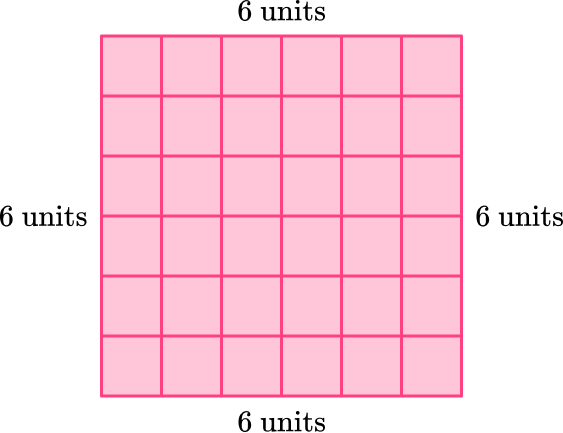To find the perimeter (the total distance around the square), add all the side lengths:

6 + 6 + 6 + 6 = 24

You can also multiply one side length by 4 to calculate the perimeter:

4 \times 6=24.

The perimeter of the square is 24 units.

2) Which square has a perimeter of 8 units?Since the perimeter is 8 units, the sum of the lengths of all the sides is 8 .

That means the length of each side of the square must be 2 units, since

2 + 2 + 2 + 2 = 8.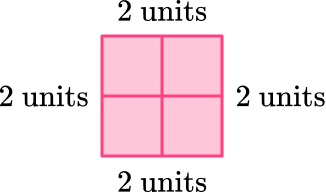You can also think about what number times 4 is equal to 8 : 4 \, \times \, ?= 8.

Since 4 \times 2= 8, the square has side lengths of 2 units.

3) What is the perimeter of the square?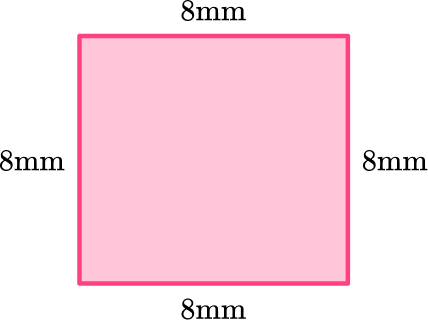8 \, mm32 \, mm64 \, mm28 \, mmThe sides are all equal lengths, so to calculate the perimeter (the total distance around the square), add them:

8 + 8 + 8 + 8 = 32

Since each side of a square is the same, you can also multiply one side length by 4 to calculate the perimeter: 4 \times 8= 32 .

The side lengths are measured in millimeters, so the total perimeter is in millimeters.

The perimeter of the square is 32 {~mm} .

4) What is the perimeter of the square?82 \, m23 \, m46 \, m92 \, m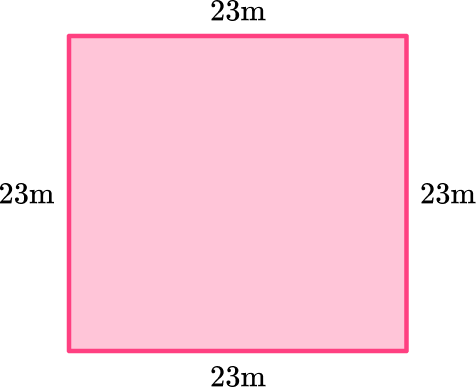The sides are all equal lengths, so to calculate the perimeter (the total distance around the square), add them:

23 + 23 + 23 + 23 = 92

Since each side of a square is the same, you can also multiply one side length by 4 to calculate the perimeter: 4 \times 23= 92.

The side lengths are measured in meters, so the total perimeter is in meters.

The perimeter of the square is 92 {~m} .

5) A hotel has a square pool. One side of the pool is 24 feet. What is the perimeter of the pool?

24 \, ft88 \, ft96 \, ft48 \, ft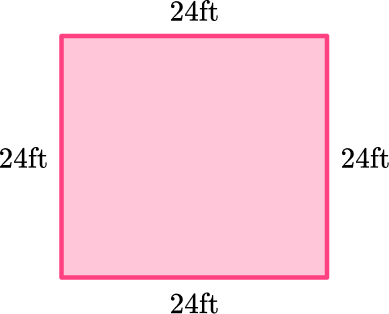The length of the side is the same, so to calculate the perimeter (the total distance around the square), add them:

24 + 24 + 24 + 24 = 96

Since each side of a square is the same, you can also multiply one side length by 4 to calculate the perimeter: 4 \times 24= 96 .

The side lengths are measured in feet, so the total perimeter is in feet.

The perimeter of the square is 96 {~ft} .

6) The perimeter of the square is 60 {~cm} . What is the length of its sides?

240 \, cm15 \, cm120 \, cm30 \, cmRemember, all the sides of the square are congruent (equal).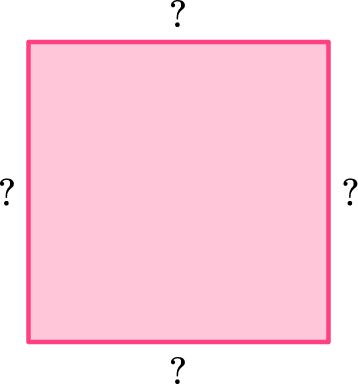So, the perimeter is the same number added 4 times:

? \; + \; ? \; + \; ? \; + \; ? = 60 {~cm}

Another way to think about it is ? \times 4= 60.

Since 15 + 15 + 15 + 15= 60 and 15 \times 4= 60 , the side length of the square is 15.

The square is measured in centimeters (cm) .

The missing side length is 15 {~cm} .

## Perimeter of a square FAQs

How is the area of a square connected to the perimeter?

Perimeter is the total length around a square and is measured in units. Area of the square is the space within a rectangle and is measured with square units. They represent different parts of a square, but both require knowing the dimensions of a side to solve.

How do you find the perimeter of other quadrilaterals?

Just like with squares, you add the 4 side lengths up to find the total distance around the quadrilateral. However, unless the shape is a square or a rhombus, not all sides will be equal. The perimeter of rectangles, parallelograms, and trapezoids can have different side lengths.

## Still stuck?

At Third Space Learning, we specialize in helping teachers and school leaders to provide personalized math support for more of their students through high-quality, online one-on-one math tutoring delivered by subject experts.

Each week, our tutors support thousands of students who are at risk of not meeting their grade-level expectations, and help accelerate their progress and boost their confidence.